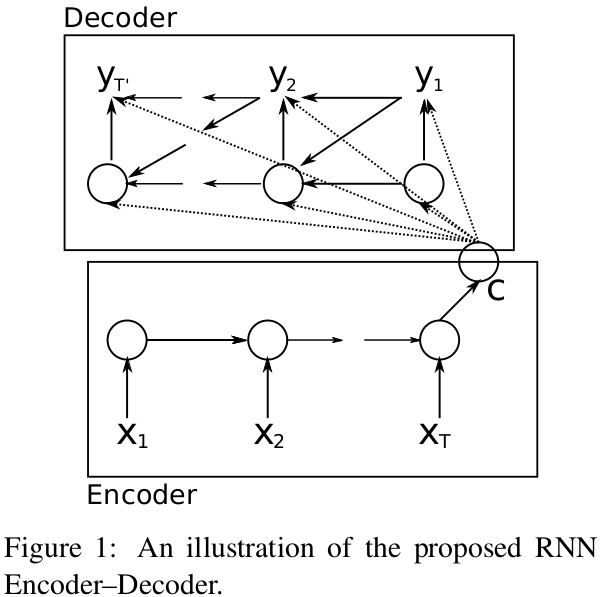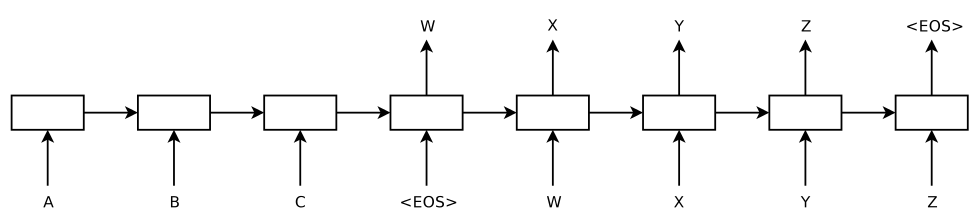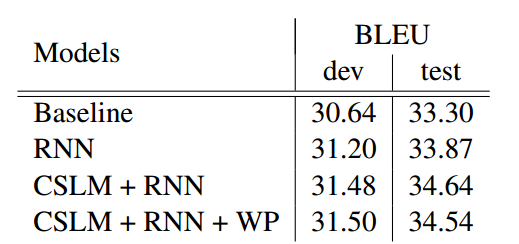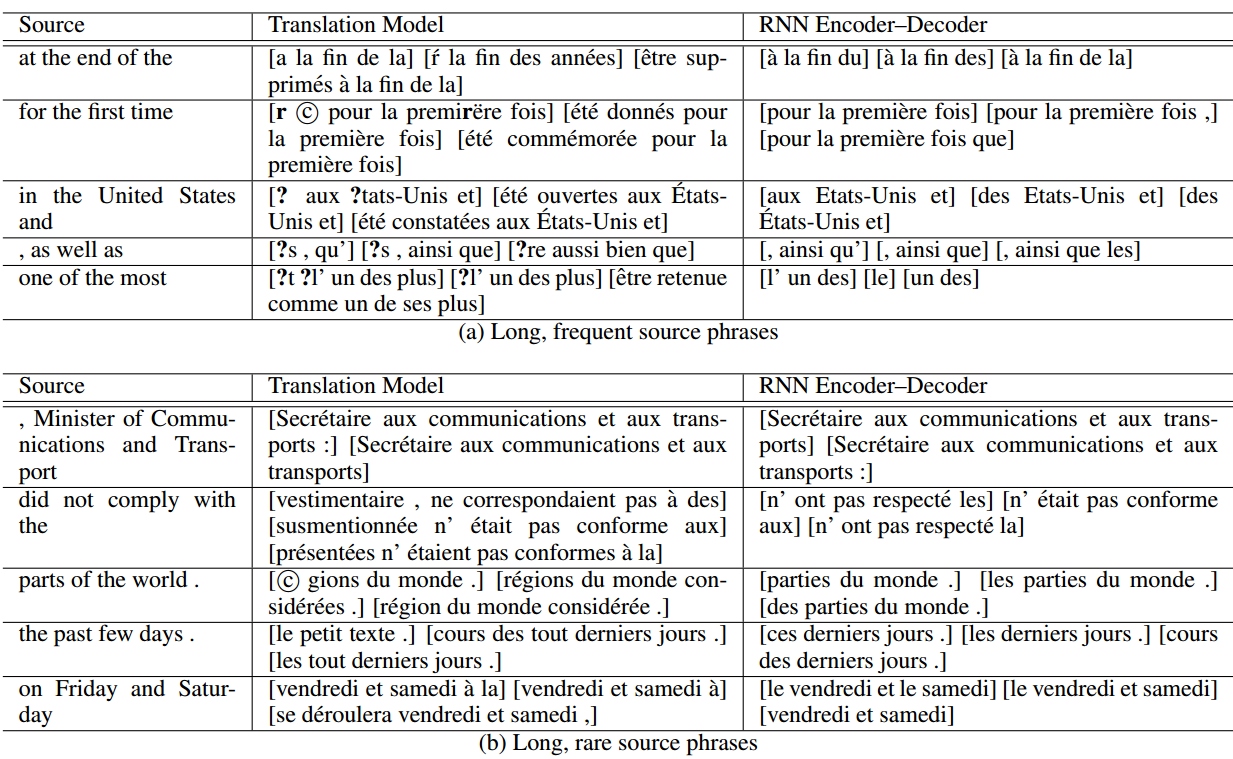# 论文笔记：Learning Phrase Representation using RNN Encode-Decoder for Statistical Machine Translation"Sequence to Sequence" 这篇论文的作者自己也表示 "Our approach is very similar to Cho et al" 。

$P(y_{t})=f(h_{t}, y_{t-1})$

$P(y_{t})=f(h_{t}, y_{t-1}, c)$

Cho 的 Encoder-Decoder 模型中，Encoder 的行为比较简单，重点在 Decoder 上。

Decoder 中 t 时刻的内部状态的 $$h_{t}$$ 为:

$h_{t}=g(h_{t-1}, y_{t-1}, c)$

$P(y_{t}|y_{t-1}, y_{t-2},...,y_{1}, c)=f(h_{t}, y_{t-1}, c)$

$\arg\max_{\theta}\frac{1}{N}\sum_{n=1}^{N}logP_{\theta}(Y_{n}|X_{n})$

1. Baseline configuration
2. Baseline + RNN
3. Baseline + CSLM + RNN
4. Baseline + CSLM + RNN + Word penaltyphrase pair 打分的结果如下: